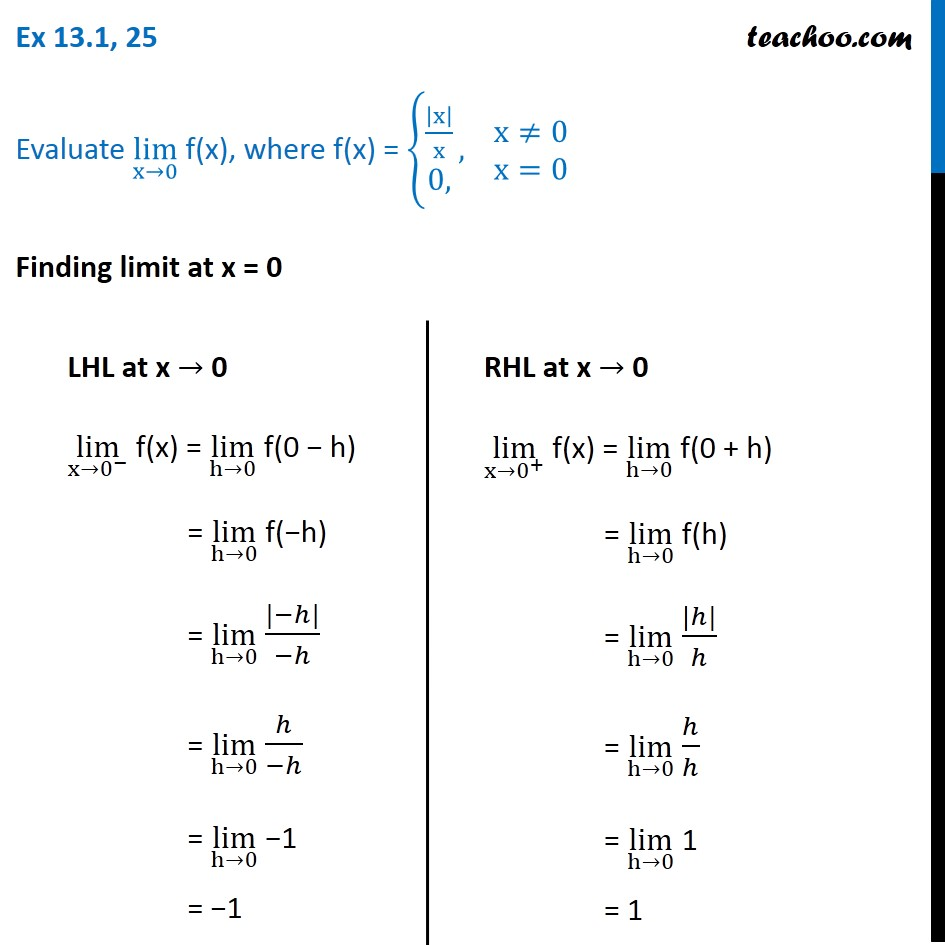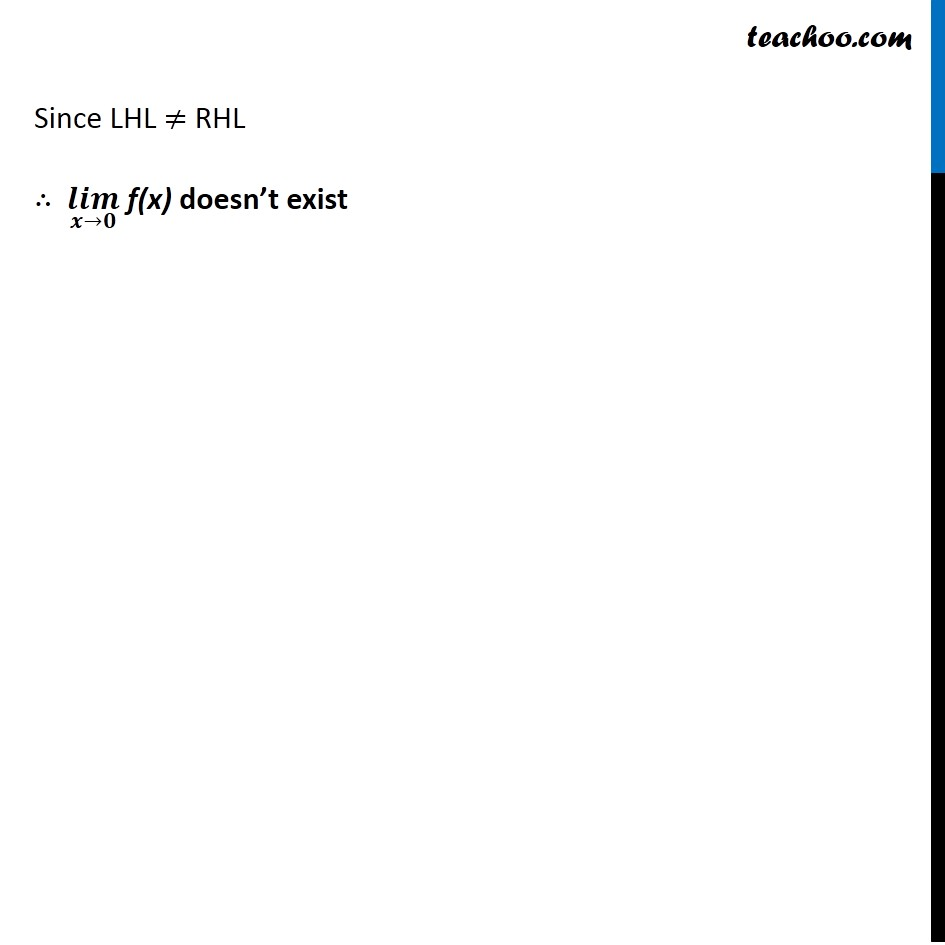1. Chapter 13 Class 11 Limits and Derivatives
2. Concept wise
3. Limits - Limit exists

Transcript

Ex 13.1, 25 Evaluate lim┬(x→0) f(x), where f(x) = {█(|x|/x@0,)┤, ■8(x≠0@x=0) Finding limit at x = 0 LHL at x → 0 lim┬(x→0^− ) f(x) = lim┬(h→0) f(0 − h) = lim┬(h→0) f(−h) = lim┬(h→0) (|−ℎ|)/(−ℎ) = lim┬(h→0) ℎ/(−ℎ) = lim┬(h→0) −1 = −1 RHL at x → 0 lim┬(x→0^+ ) f(x) = lim┬(h→0) f(0 + h) = lim┬(h→0) f(h) = lim┬(h→0) (|ℎ|)/ℎ = lim┬(h→0) ℎ/ℎ = lim┬(h→0) 1 = 1 Since LHL ≠ RHL ∴ (𝒍𝒊𝒎)┬(𝒙→𝟎) f(x) doesn’t exist

Limits - Limit exists